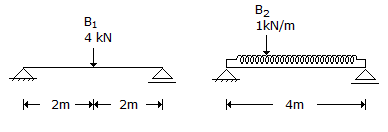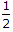# Civil Engineering - UPSC Civil Service Exam Questions

26.

Consider the following statements :
Kiln seasoning of timber results is :
1. reduced density.
2. reduced life.
3. dimensional stability.
Which of the following given above is/are correct ?

 A. 1, 2 and 3 B. 1 only C. 2 and 3 D. 1 and 3

Explanation:

No answer description available for this question. Let us discuss.

27.

The approximate ratio of strength of 15 cm x 30 cm concrete cylinder to that of 15 cm cube of the same concrete is

 A. 1.25 B. 1 C. 0.85 D. 0.5

Explanation:

No answer description available for this question. Let us discuss.

28.

Consider the following characteristic of soil layer :
1. Poisson's ratio
2. Young's modulus
3. Finite nature of soil layer
4. Effect of water table
5. Rigidity of footing
Westergaard's analysis for pressure distribution in soils utilises

 A. 1, 3, 4 and 5 B. 2, 3, 4 and 5 C. 3, 4 and 5 D. 1 and 2

Explanation:

No answer description available for this question. Let us discuss.

29.

Consider the following statements associated with the laws of weights in the theory of erros :
1. If an equation is multiplied by its own weight, then the weight of the resulting equation is equal to the reciprocal of the weight of the equation.
2. The weight of the algebraic sum of two or more quantities is equal to the reciprocal of the sum of the individual weights.
3. If the quantity of a given weight is multiplied by a factor, then the weight of the result is obtained by dividing its given weight by the square root of that factor.
4. If the quantity of a given weight is divided by a factor, then the weight of the result is obtained by multiplying its given weight by the square of that factor.
Of these statements :

 A. 1 and 4 are correct B. 2 and 3 are correct C. 3 and 4 are correct D. 1 and 3 are correct

Explanation:

No answer description available for this question. Let us discuss.

30.

The given diagrams show the details of two simply supported beams B1 and B2. EI is constant throughout the length and same for both the beams. The beams have the same area of cross-section and the same depth. What is the ratio of maximum bending stress in B2 to that in B1 ?A. 4 B. 1 C. 2 D.